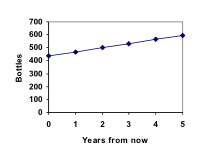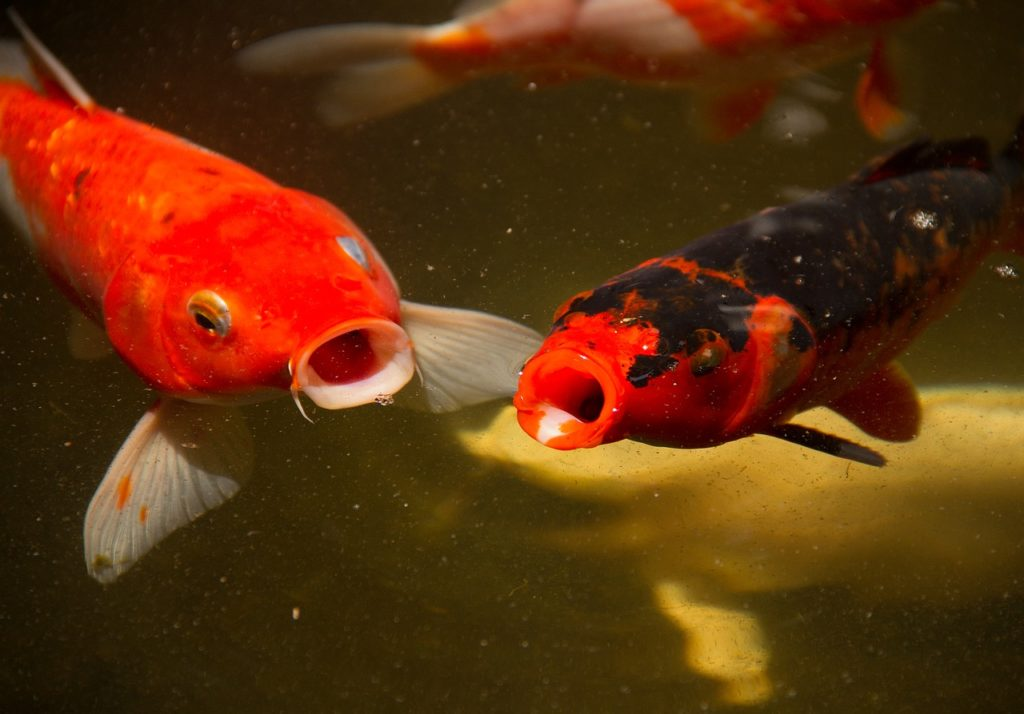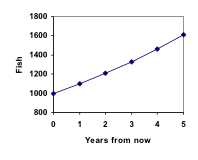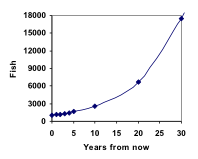## Linear and Geometric Growth

### Learning Outcomes

• Determine whether data or a scenario describe linear or geometric growth
• Identify growth rates, initial values, or point values expressed verbally, graphically, or numerically, and translate them into a format usable in calculation
• Calculate recursive and explicit equations for linear and geometric growth given sufficient information, and use those equations to make predictions

Having a constant rate of change is the defining characteristic of linear growth. Plotting coordinate pairs associated with constant change will result in a straight line, the shape of linear growth. In this section, we will formalize a way to describe linear growth using mathematical terms and concepts. By the end of this section, you will be able to write both a recursive and explicit equations for linear growth given starting conditions, or a constant of change.  You will also be able to recognize the difference between linear and geometric growth given a graph or an equation.

## Linear (Algebraic) Growth

### Predicting Growth

Marco is a collector of antique soda bottles. His collection currently contains 437 bottles. Every year, he budgets enough money to buy 32 new bottles. Can we determine how many bottles he will have in 5 years, and how long it will take for his collection to reach 1000 bottles?

While you could probably solve both of these questions without an equation or formal mathematics, we are going to formalize our approach to this problem to provide a means to answer more complicated questions.

Suppose that n represents the number, or population, of bottles Marco has after n years. So 0 would represent the number of bottles now, 1 would represent the number of bottles after 1 year, 2 would represent the number of bottles after 2 years, and so on. We could describe how Marco’s bottle collection is changing using:

0 = 437

n = P­n-1 + 32

This is called a recursive relationship. A recursive relationship is a formula which relates the next value in a sequence to the previous values. Here, the number of bottles in year n can be found by adding 32 to the number of bottles in the previous year, n-1. Using this relationship, we could calculate:

1 = P­0 + 32 = 437 + 32 = 469

2 = P­1 + 32 = 469 + 32 = 501

P3 = P­2 + 32 = 501 + 32 = 533

4 = P­3 + 32 = 533 + 32 = 565

5 = P­4 + 32 = 565 + 32 = 597

We have answered the question of how many bottles Marco will have in 5 years.However, solving how long it will take for his collection to reach 1000 bottles would require a lot more calculations.

While recursive relationships are excellent for describing simply and cleanly how a quantity is changing, they are not convenient for making predictions or solving problems that stretch far into the future. For that, a closed or explicit form for the relationship is preferred. An explicit equation allows us to calculate n directly, without needing to know P­n-1. While you may already be able to guess the explicit equation, let us derive it from the recursive formula. We can do so by selectively not simplifying as we go:

1 = 437 + 32                                        = 437 + 1(32)

2 = P­1 + 32 = 437 + 32 + 32             = 437 + 2(32)

P3 = P­2 + 32 = (437 + 2(32)) + 32     = 437 + 3(32)

4 = P­3 + 32 = (437 + 3(32)) + 32     = 437 + 4(32)

You can probably see the pattern now, and generalize that

P­n = 437 + n(32) = 437 + 32n

Using this equation, we can calculate how many bottles he’ll have after 5 years:

5 = 437 + 32(5) = 437 + 160 = 597

We can now also solve for when the collection will reach 1000 bottles by substituting in 1000 for n and solving for n

1000 = 437 + 32n

563 = 32n

n = 563/32 = 17.59

So Marco will reach 1000 bottles in 18 years.

The steps of determining the formula and solving the problem of Marco’s bottle collection are explained in detail in the following videos.

In this example, Marco’s collection grew by the same number of bottles every year. This constant change is the defining characteristic of linear growth. Plotting the values we calculated for Marco’s collection, we can see the values form a straight line, the shape of linear growth.

### Linear Growth

If a quantity starts at size 0 and grows by d every time period, then the quantity after n time periods can be determined using either of these relations:

n = P­n-1 + d

#### Explicit form

n = P­0 + d n

In this equation, d represents the common difference – the amount that the population changes each time n increases by 1.

### Connection to Prior Learning: Slope and Intercept

You may recognize the common difference, d, in our linear equation as slope. In fact, the entire explicit equation should look familiar – it is the same linear equation you learned in algebra, probably stated as y = mx + b.

In the standard algebraic equation y = mx + b, b was the y-intercept, or the y value when x was zero. In the form of the equation we’re using, we are using P­0­ to represent that initial amount.

In the y = mx + b equation, recall that m was the slope. You might remember this as “rise over run,” or the change in y divided by the change in x. Either way, it represents the same thing as the common difference, d, we are using – the amount the output Pn changes when the input n increases by 1.

The equations y = mx + b and n = P­0 + d n mean the same thing and can be used the same ways. We’re just writing it somewhat differently.

### Examples

The population of elk in a national forest was measured to be 12,000 in 2003, and was measured again to be 15,000 in 2007. If the population continues to grow linearly at this rate, what will the elk population be in 2014?

Gasoline consumption in the US has been increasing steadily. Consumption data from 1992 to 2004 is shown below. Find a model for this data, and use it to predict consumption in 2016. If the trend continues, when will consumption reach 200 billion gallons?

 Year ’92 ’93 ’94 ’95 ’96 ’97 ’98 ’99 ’00 ’01 ’02 ’03 ’04 Consumption (billion of gallons) 110 111 113 116 118 119 123 125 126 128 131 133 136

The steps for reaching this answer are detailed in the following video.

The cost, in dollars, of a gym membership for n months can be described by the explicit equation n = 70 + 30n. What does this equation tell us?

The explanation for this example is detailed below.

### Try It

The number of stay-at-home fathers in Canada has been growing steadily. While the trend is not perfectly linear, it is fairly linear. Use the data from 1976 and 2010 to find an explicit formula for the number of stay-at-home fathers, then use it to predict the number in 2020.

 Year 1976 1984 1991 2000 2010 # of Stay -at-home fathers 20610 28725 43530 47665 53555

### When Good Models Go Bad

When using mathematical models to predict future behavior, it is important to keep in mind that very few trends will continue indefinitely.

### Example

Suppose a four year old boy is currently 39 inches tall, and you are told to expect him to grow 2.5 inches a year.

We can set up a growth model, with n = 0 corresponding to 4 years old.

#### Recursive form

0 = 39

P­n = P­n-1 + 2.5

#### Explicit form

n = 39 + 2.5(n)

So at 6 years old, we would expect him to be

2 = 39 + 2.5(2) = 44 inches tall

Any mathematical model will break down eventually. Certainly, we shouldn’t expect this boy to continue to grow at the same rate all his life. If he did, at age 50 he would be

P­46 = 39 + 2.5(46) = 154 inches tall = 12.8 feet tall!

When using any mathematical model, we have to consider which inputs are reasonable to use. Whenever we extrapolate, or make predictions into the future, we are assuming the model will continue to be valid.

View a video explanation of this breakdown of the linear growth model here.

## Exponential (Geometric ) Growth

### Population Growth

Suppose that every year, only 10% of the fish in a lake have surviving offspring. If there were 100 fish in the lake last year, there would now be 110 fish. If there were 1000 fish in the lake last year, there would now be 1100 fish. Absent any inhibiting factors, populations of people and animals tend to grow by a percent of the existing population each year.Suppose our lake began with 1000 fish, and 10% of the fish have surviving offspring each year. Since we start with 1000 fish, 0 = 1000. How do we calculate 1? The new population will be the old population, plus an additional 10%. Symbolically:

1 = 0 + 0.10P­0

Notice this could be condensed to a shorter form by factoring:

P­1 = 0 + 0.100 = 10 + 0.10P­0 = (1+ 0.10)0 = 1.100

While 10% is the growth rate, 1.10 is the growth multiplier. Notice that 1.10 can be thought of as “the original 100% plus an additional 10%.”

For our fish population,

1 = 1.10(1000) = 1100

We could then calculate the population in later years:

2 = 1.101 = 1.10(1100) = 1210

P­3 = 1.10P2 = 1.10(1210) = 1331

Notice that in the first year, the population grew by 100 fish; in the second year, the population grew by 110 fish; and in the third year the population grew by 121 fish.

While there is a constant percentage growth, the actual increase in number of fish is increasing each year.

Graphing these values we see that this growth doesn’t quite appear linear.A walkthrough of this fish scenario can be viewed here:

To get a better picture of how this percentage-based growth affects things, we need an explicit form, so we can quickly calculate values further out in the future.

Like we did for the linear model, we will start building from the recursive equation:

1 = 1.10(0 )= 1.10(1000)

2 = 1.10(1 )= 1.10(1.10(1000)) = 1.102(1000)

3 = 1.10(2 )= 1.10(1.102(1000)) = 1.103(1000)

4 = 1.10(3 )= 1.10(1.103(1000)) = 1.104(1000)

Observing a pattern, we can generalize the explicit form to be:

n = 1.10n(1000), or equivalently, n = 1000(1.10n)

From this, we can quickly calculate the number of fish in 10, 20, or 30 years:10 = 1.1010(1000) = 2594

20 = 1.1020(1000) = 6727

30 = 1.1030(1000) = 17449

Adding these values to our graph reveals a shape that is definitely not linear. If our fish population had been growing linearly, by 100 fish each year, the population would have only reached 4000 in 30 years, compared to almost 18,000 with this percent-based growth, called exponential growth.

A video demonstrating the explicit model of this fish story can be viewed here:

In exponential growth, the population grows proportional to the size of the population, so as the population gets larger, the same percent growth will yield a larger numeric growth.

### Exponential Growth

If a quantity starts at size 0 and grows by R% (written as a decimal, r) every time period, then the quantity after n time periods can be determined using either of these relations:

n = (1+r) n-1

#### Explicit form

n = (1+r)n 0         or equivalently, P­n = 0 (1+r)n

We call r the growth rate.

The term (1+r) is called the growth multiplier, or common ratio.

### Example

Between 2007 and 2008, Olympia, WA grew almost 3% to a population of 245 thousand people. If this growth rate was to continue, what would the population of Olympia be in 2014?

The following video explains this example in detail.

### Evaluating exponents on the calculator

To evaluate expressions like (1.03)6, it will be easier to use a calculator than multiply 1.03 by itself six times. Most scientific calculators have a button for exponents.  It is typically either labeled like:

^ ,   yx ,   or xy .

To evaluate 1.036 we’d type 1.03 ^ 6, or 1.03 yx 6.  Try it out – you should get an answer around 1.1940523.

### Try It

India is the second most populous country in the world, with a population in 2008 of about 1.14 billion people. The population is growing by about 1.34% each year. If this trend continues, what will India’s population grow to by 2020?

### Examples

A friend is using the equation n = 4600(1.072)n to predict the annual tuition at a local college. She says the formula is based on years after 2010. What does this equation tell us?

View the following to see this example worked out.

In 1990, residential energy use in the US was responsible for 962 million metric tons of carbon dioxide emissions. By the year 2000, that number had risen to 1182 million metric tons. If the emissions grow exponentially and continue at the same rate, what will the emissions grow to by 2050?

### Rounding

As a note on rounding, notice that if we had rounded the growth rate to 2.1%, our calculation for the emissions in 2050 would have been 3347.   Rounding to 2% would have changed our result to 3156. A very small difference in the growth rates gets magnified greatly in exponential growth. For this reason, it is recommended to round the growth rate as little as possible.

If you need to round, keep at least three significant digits – numbers after any leading zeros.   So 0.4162 could be reasonably rounded to 0.416. A growth rate of 0.001027 could be reasonably rounded to 0.00103.

### Evaluating roots on the calculator

In the previous example, we had to calculate the 10th root of a number. This is different than taking the basic square root, √. Many scientific calculators have a button for general roots.  It is typically labeled like:

$\sqrt[y]{x}$

To evaluate the 3rd root of 8, for example, we’d either type 3 $\sqrt[x]{{}}$ 8, or 8 $\sqrt[x]{{}}$ 3, depending on the calculator. Try it on yours to see which to use – you should get an answer of 2.

If your calculator does not have a general root button, all is not lost. You can instead use the property of exponents which states that:

$\sqrt[n]{a}={a}^{\frac{1}{2}}$.

So, to compute the 3rd root of 8, you could use your calculator’s exponent key to evaluate 81/3. To do this, type:

8 yx ( 1 ÷ 3 )

The parentheses tell the calculator to divide 1/3 before doing the exponent.

### Try It

The number of users on a social networking site was 45 thousand in February when they officially went public, and grew to 60 thousand by October. If the site is growing exponentially, and growth continues at the same rate, how many users should they expect two years after they went public?

### Example

Looking back at the last example, for the sake of comparison, what would the carbon emissions be in 2050 if emissions grow linearly at the same rate?

A demonstration of this example can be seen in the following video.

So how do we know which growth model to use when working with data? There are two approaches which should be used together whenever possible:

1. Find more than two pieces of data. Plot the values, and look for a trend. Does the data appear to be changing like a line, or do the values appear to be curving upwards?
2. Consider the factors contributing to the data. Are they things you would expect to change linearly or exponentially? For example, in the case of carbon emissions, we could expect that, absent other factors, they would be tied closely to population values, which tend to change exponentially.